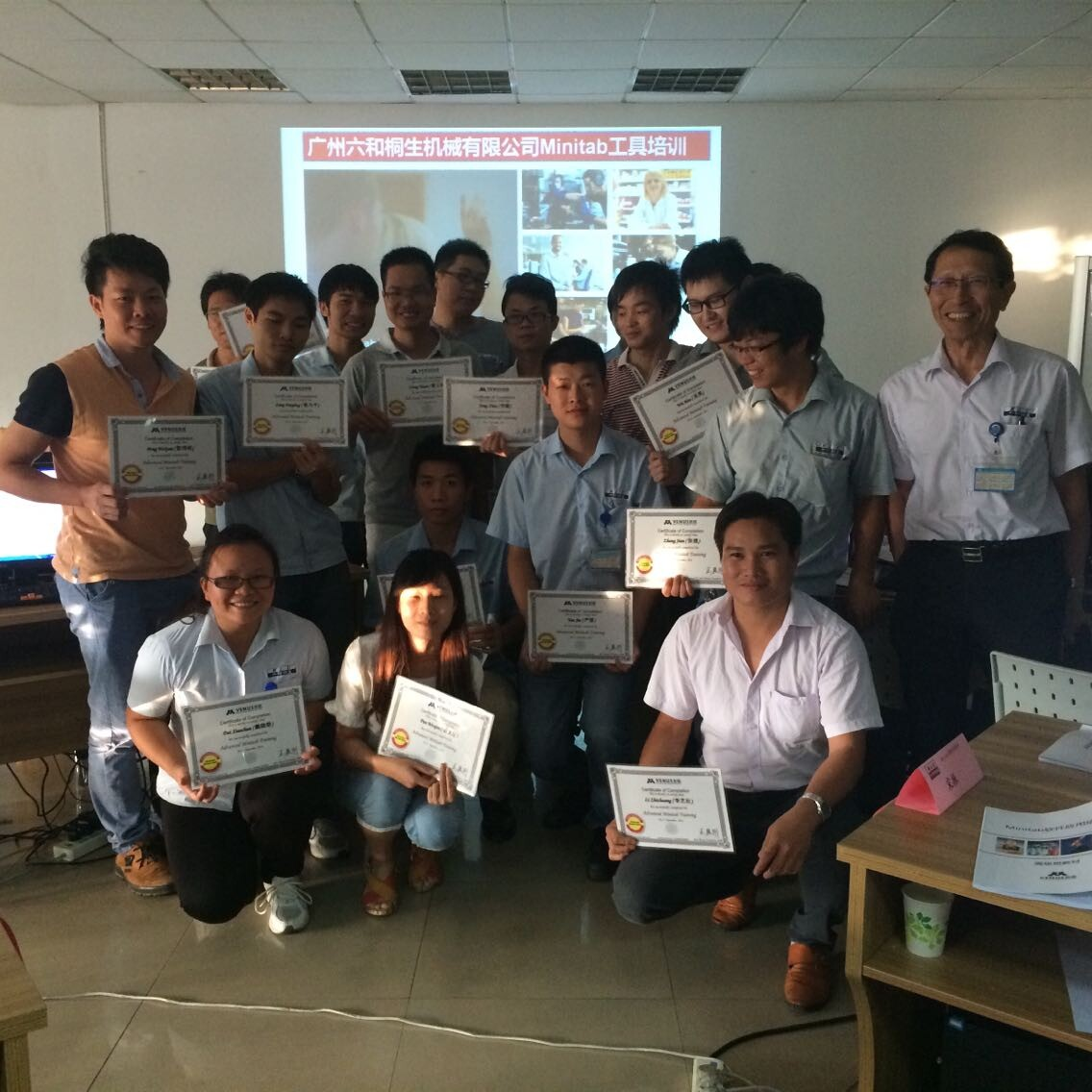CoursesContact： Phenix Zhu Tel： +86-21-33729081 Email： info@venusquality.com

Current location：Home > Courses > Advanced Minitab Course

Description:

This course covers Minitab software's approach to data manipulation and analysis, measurement systems analysis, design of experiments, and statistical process control in direct support of DMAIC breakthrough projects.

Duration:

Two(2)days

Benefits:

Learn how to

import and manipulate data

perform data analysis for a Six Sigma projects

design and analyze an experiment

create control charts.

Who Should Attend:

Six Sigma professionals, Six Sigma team members

Key Topics:

• Type of Minitab Windows
• Work Sheet
• Generate Normal Data
• Make Patterned Data (Text, data)
• Graph functions
• Scatter plot
• Histogram
• Probability plot
• Probability Distribution Plot
• Basic Statistic Overview
• 1 sample Z-test and confidence interval
• 1 sample t-test
• 2 sample t-test
• Paired t-test
• F-test
• 1 test and confidence interval of a proportion
• 2 test and confidence interval of two proportion
• Multi-Vari Analyze
• Main Effect Plot
• Multi-vari chart
• One way ANOVA
• Basic of Hypothesis test
• One way ANOVA (Stacked)
• One way ANOVA (unstuck)
• Graph &Compare
• Two way ANOVA
• Balance ANOVA
• General linear fully nested ANOVA
• Correlation and Regression
• Correlation Analysis
• Regression
• Step wise regression
• Best fits regression
• Full factorial DOE
• Basic of DOE
• Design of Experiment
• Center Point& Blocking
• Response Surface Designs Overview
• 3k Factorial Designs
• Response Surface Designs (Box-Behnken Designs, Central Composite Designs)
• Fractional Factorial DOE
• Reliability Analyze
• Basic of Reliability Analysis
• Estimate test plan
• Accelerated life test plan
• Distribution analyze (right censoringweixin：venusgroup